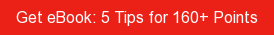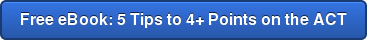# SAT / ACT Prep Online Guides and TipsMost likely, during high school you've taken a mix of classes: some honors, some standard, and some APs. The weighted GPA reflects that those classes have different difficulty levels. So what's the easiest way to figure out what your weighted GPA is? We have two great methods to calculate it!

## What Is a Weighted GPA?

Your GPA, or grade point average, is a way for colleges to quickly see a solid, summary indicator of your intelligence, work ethic, willingness to challenge yourself, and skills.

A weighted GPA showcases the hard work and challenge of your high school career by reflecting whether the classes you took were standard level, honors level, or AP/IB level. It does this by adding .5 to every honors class GPA conversion decimal and adding 1 to every AP class conversion decimal, creating a scale that goes from 0.0 to 5.0.

So, for example, imagine Diane gets an A in AP French and Sven gets an A in standard level Geography. A weighted GPA would recognize that the AP class was most likely harder, so Diane’s A would become 5.0, while Sven's A would be 4.0.

Here is a table that explains how all this usually works in more detail:

 Letter Grade Percentile Standard GPA Honors GPA AP/IB GPA A+ 97-100 4.0 4.5 5.0 A 93-96 4.0 4.5 5.0 A- 90-92 3.7 4.2 4.7 B+ 87-89 3.3 3.8 4.3 B 83-86 3.0 3.5 4.0 B- 80-82 2.7 3.2 3.7 C+ 77-79 2.3 2.8 3.3 C 73-76 2.0 2.5 3.0 C- 70-72 1.7 2.2 2.7 D+ 67-69 1.3 1.8 2.3 D 65-66 1.0 1.5 2.0 F Below 65 0.0 0.0 0.0

## How Do You Calculate Your Weighted GPA?

There are two different ways to calculate your weighted GPA.

### Class-by-class method

This method goes through each of the classes that you've taken, one by one:

#1: First, convert all the final class grades you’ve gotten, keeping careful track of whether the course was honors level, AP level, or standard.

#2: Next, add up all of these converted decimals – this is your sum.

#3: Then, count the total number of classes you have taken.

#4: Finally, divide the sum by the number of classes and round to the nearest tenth—this is your weighted GPA.

Pro tip: you can't simply add each individual year's GPAs together and divide by 4 because you may have taken a different number of classes each year.

### Sorted-classes shortcut method

If you've already done an unweighted GPA calculation, then this method is for you:

#1: First, count separately the number of standard classes, honors classes, and AP classes that you've taken.

#2: Next, add your unweighted converted grade sum + (.5 * number of honors classes) + number of AP classes. This is your weighted sum.

#3: Finally, divide the weighted sum by the total number of classes you've taken.

## Step-by-Step Example Calculation

Let's go through an example of how this works in practice by calculating the weighted GPA of spymaster alias Jane Doe. We will be calculating the GPA Jane submits on her college applications, so we will leave off her senior year grades.

Note that on her transcript, classes marked with a “+” are honors classes, and those marked with an “a” are AP classes.First let’s convert her grades. I will convert them into weighted and unweighted format so you can see the difference:

 9th grade 10th grade 11th grade Unweight. Weight. Unweight. Weight. Unweight. Weight. 3.0 4.0 3.3 3.8 3.3 4.3 3.7 3.7 4.0 4.0 4.0 4.0 4.0 4.0 3.0 3.0 3.3 3.8 3.0 3.0 3.3 3.8 4.0 5.0 3.3 3.8 3.7 4.7 4.0 5.0 Total 17.0 18.5 17.3 19.3 18.6 22.1 Honors classes 2 2 1 AP classes 0 1 3 Total number of classes 6 6 6

Boy, Jane really stepped up her game junior year! Nicely done.

### Class-by-class method:

Sum of weighted scores = 59.4

Number of classes taken = 15

Jane's weighted GPA = 59.4 / 15 = 4.0

### Sorted-classes method:

Sum of unweighted scores = 52.9

Number of honors classes = 5

Number of AP classes = 4

Number of classes taken = 15

Weighted sum = 52.9 + (.5 * 5) + 4 = 59.4

Jane's weighted GPA = 59.4 / 15 = 4.0

## What’s Next?

Now that you've gone through our weighted GPA calculator, check whether Jane was right to go all out academically junior year by learning which year of high school is the most important for your college applications.

Let us help you figure out the pros and cons of weighted and unweighted GPAs with our comprehensive explanation.

Explore what a good or bad GPA score is, and how you compare to the average high school student.

Get the scoop on whether colleges use weighted or unweighted GPAs when assessing your application.

Want to improve your SAT score by 160 points or your ACT score by 4 points? We've written a guide for each test about the top 5 strategies you must be using to have a shot at improving your score. Download it for free now: